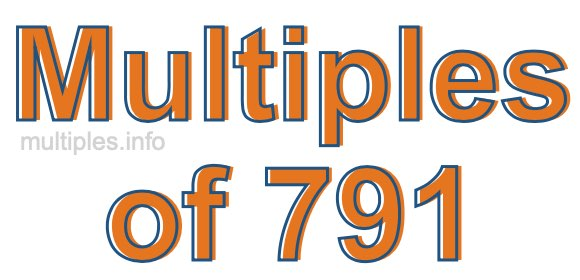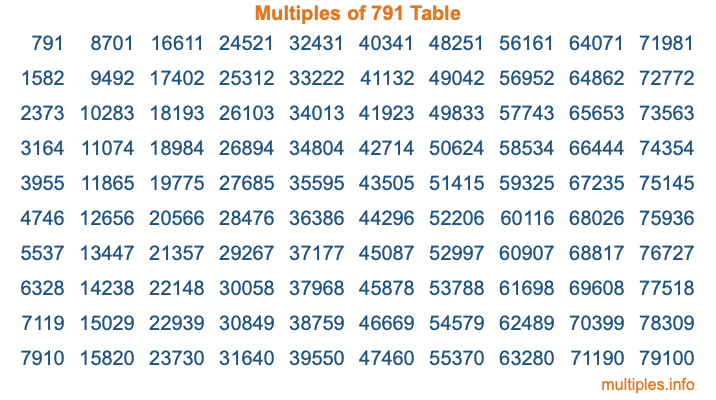Multiples of 791Welcome to the Multiples of 791 page. Here we will first teach you everything you will ever need to know about the multiples of 791, and then give you a study guide summary of everything we taught you to make sure you remember it all. Use this page to look up facts and learn information about the multiples of 791. This page will make you a multiples of seven hundred ninety-one expert!

Definition of Multiples of 791
Multiples of 791 are all the numbers that when divided by 791 equal an integer. Each of the multiples of 791 are called a multiple. A multiple of 791 is created by multiplying 791 by an integer.

Therefore, to create a list of multiples of 791, you start with 1 multiplied by 791, then 2 multiplied by 791, then 3 multiplied by 791, and so on for as long as you want. Thus, the list of the first five multiples of 791 is 791, 1582, 2373, 3164, and 3955. To see a larger list of multiples of 791, see the printable image of Multiples of 791 further down on this page. We also have a category where you can choose any nth multiple of 791.

Multiples of 791 Checker
The Multiples of 791 Checker below checks to see if any number of your choice is a multiple of 791. In other words, it checks to see if there is any number (integer) that when multiplied by 791 will equal your number. To do that, we divide your number by 791. If the the quotient is an integer, then your number is a multiple of 791.

Is  a multiple of 791?

Least Common Multiple of 791 and ...
A Least Common Multiple (LCM) is the lowest multiple that two or more numbers have in common. This is also called the smallest common multiple or lowest common multiple and is useful to know when you are adding our subtracting fractions. Enter one or more numbers below (791 is already entered) to find the LCM.

Check out our LCM Calculator if you need more details about the Least Common Multiple or if you need the LCM for different numbers for adding and subtraction fractions.

nth Multiple of 791
As we stated above, 791 is the first multiple of 791, 1582 is the second multiple of 791, 2373 is the third multiple of 791, and so on. Enter a number below to find the nth multiple of 791.

th multiple of 791

Multiples of 791 vs Factors of 791
791 is a multiple of 791 and a factor of 791, but that is where the similarities end. All postive multiples of 791 are 791 or greater than 791. All positive factors of 791 are 791 or less than 791.

Below is the beginning list of multiples of 791 and the factors of 791 so you can compare:

Multiples of 791: 791, 1582, 2373, 3164, 3955, etc.

Factors of 791: 1, 7, 113, 791

As you can see, the multiples of 791 are all the numbers that you can divide by 791 to get a whole number. The factors of 791, on the other hand, are all the whole numbers that you can multiply by another whole number to get 791.

It's also interesting to note that if a number (x) is a factor of 791, then 791 will also be a multiple of that number (x).

Multiples of 791 vs Divisors of 791
The divisors of 791 are all the integers that 791 can be divided by evenly. Below is a list of the divisors of 791.

Divisors of 791: 1, 7, 113, 791

The interesting thing to note here is that if you take any multiple of 791 and divide it by a divisor of 791, you will see that the quotient is an integer.

Multiples of 791 Table
Below is an image of the first 100 multiples of 791 in a table. The table is in chronological order, column by column. The first column has the first ten multiples of 791, the second column has the next ten multiples of 791, and so on.The Multiples of 791 Table is also referred to as the 791 Times Table or Times Table of 791. You are welcome to print out our table for your studies.

Negative Multiples of 791
Although not often discussed or needed in math, it is worth mentioning that you can make a list of negative multiples of 791 by multiplying 791 by -1, then by -2, then by -3, and so on, to get the following list of negative multiples of 791:

-791, -1582, -2373, -3164, -3955, etc.

Multiples of 791 Summary
Below is a summary of important Multiples of 791 facts that we have discussed on this page. To retain the knowledge on this page, we recommend that you read through the summary and explain to yourself or a study partner why they hold true.

There are an infinite number of multiples of 791.

A multiple of 791 divided by 791 will equal a whole number.

791 divided by a factor of 791 equals a divisor of 791.

The nth multiple of 791 is n times 791.

The largest factor of 791 is equal to the first positive multiple of 791.

791 is a multiple of every factor of 791.

791 is a multiple of 791.

A multiple of 791 divided by a divisor of 791 equals an integer.

791 divided by a divisor of 791 equals a factor of 791.

Any integer times 791 will equal a multiple of 791.

Multiples of a Number
Here you can get the multiples of another number, all with the same attention to detail as we did for multiples of 791 on this page.

Multiples of
Multiples of 792
Did you find our page about multiples of seven hundred ninety-one educational? Do you want more knowledge? Check out the multiples of the next number on our list!